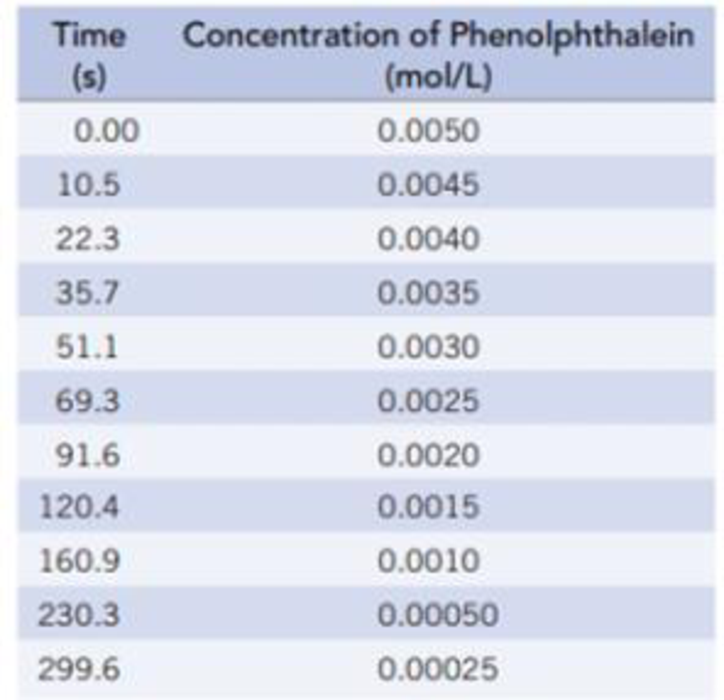Chapter 14, Problem 85IL

Chapter
Section
Textbook Problem

The color change accompanying the reaction of phenolphthalein with strong base is illustrated below. The change in concentration of the dye can be followed by spectrophotometry (Section 4.9), and some data collected by that approach are given below. The initial concentrations were [phenolphthalein] = 0.0050 mol/L and [OH‒] = 0.61 mol/L. (Data are taken from review materials for kinetics at chemed.chem.purdue.edu.) (For more details on this reaction see L Nicholson, Journal of Chemical Education, Vol. 66, p. 725, 1989.)(a) Plot the data above as [phenolphthalein] versus time, and determine the average rate from t = 0 to t = 15 seconds and from t = 100 seconds to t = 125 seconds. Does the rate change? If so, why?(b) Use a graphical method to determine the order of the reaction with respect to phenolphthalein. Write the rate law, and determine the rate constant.(c) What is the half-life for the reaction?

(a)

Interpretation Introduction

Interpretation:

The graph plotted between [Phenolphthalein] and time has to be plotted. The average rate for t=0 to t=15 and for t=100 to t=125 have to be determined.  To check whether the average rate for t=0 to t=15 and for t=100 to t=125 changes or not. The reason for difference in rates has to be explained.

Concept Introduction:

The rate of a reaction is defined by the change in concentration of substrate (reactant) or target (product) with change in time.

Rate=Concentrationchange(inreactantorproduct)Timechange

Explanation

The given information is as follows:

Time(s)[Phenolphthalein]0.0010.522.335.751.169.391.6120.4160.9230.3299.60.00500.00450.00400.00350.00300

(b)

Interpretation Introduction

Interpretation:

Using graphical method, the order of reaction with respect to phenolphthalein has to be determined. The rate law and the rate constant have to be determined.

Concept Introduction:

Order of a reaction: Order of a reaction is defined as the sum of exponents of the concentrations in the rate law of the given reaction.

For a reaction,

• If the plot drawn between concentration of reactant and time is linear, then it is a zeroth order reaction
• If the plot drawn between ln[reactant] and time is linear, then it is a first order reaction
• If the plot drawn between 1/[reactant] and time is linear, then it is a second order reaction
• For the zeroth and first order reaction, the graph has a negative slope whereas the graph has a positive slope for a second order reaction.

The rate of the reaction is equal to the slope of the reaction

Rate law or rate equation: Rate law:

It is generally the rate equation that consists of the reaction rate with the concentration or the pressures of the reactants and constant parameters.

aA + bBxXRate of reaction = k [A]m[B]n

(c)

Interpretation Introduction

Interpretation:

The half–life period for the reaction has to be determined.

Concept Introduction:

Half–lifet1/2: The half–life period is defined as the time required by the reactant to reduce its concentration to one half of its initial concentration.

The half–life period for first order reaction is as follows:

t1/2=0.693kWhere,k = Rate constantt1/2= half-life

Still sussing out bartleby?

Check out a sample textbook solution.

See a sample solution

The Solution to Your Study Problems

Bartleby provides explanations to thousands of textbook problems written by our experts, many with advanced degrees!

Get Started

Find more solutions based on key concepts